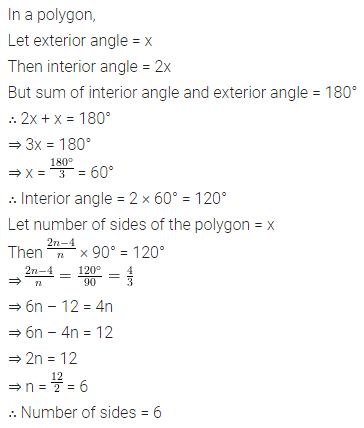# ML Aggarwal Class 8 Solutions for ICSE Maths Chapter 13 Understanding Quadrilaterals Ex 13.1

## ML Aggarwal Class 8 Solutions for ICSE Maths Chapter 13 Understanding Quadrilaterals Ex 13.1

Question 1.
Some figures are given below.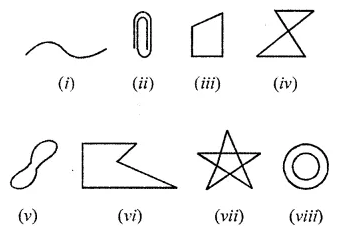Classify each of them on the basis of the following:
(a) Simple curve
(b) Simple closed curve
(c) Polygon
(d) Convex polygon
(e) Concave polygon
Solution: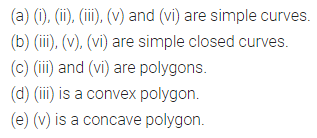Question 2.
How many diagonals does each of the following have?
(b) A regular hexagon
Solution:
(a) A convex quadrilateral: It has two diagonals.
(b) A regular hexagon: It has 9 diagonals as shown.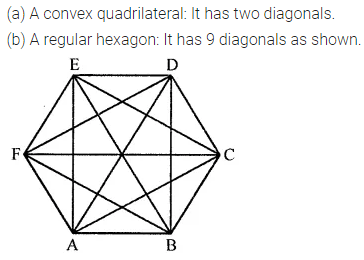Question 3.
Find the sum of measures of all interior angles of a polygon with the number of sides:
(i) 8
(ii) 12
Solution:Question 4.
Find the number of sides of a regular polygon whose each exterior angles has a measure of
(i) 24°
(ii) 60°
(iii) 72°
Solution:Question 5.
Find the number of sides of a regular polygon if each of its interior angles is
(i) 90°
(ii) 108°
(iii) 165°
Solution: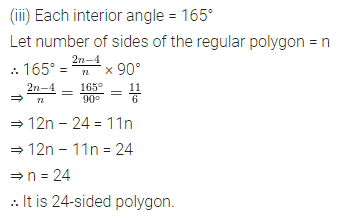Question 6.
Find the number of sides in a polygon if the sum of its interior angles is:
(i) 1260°
(ii) 1980°
(iii) 3420°
Solution: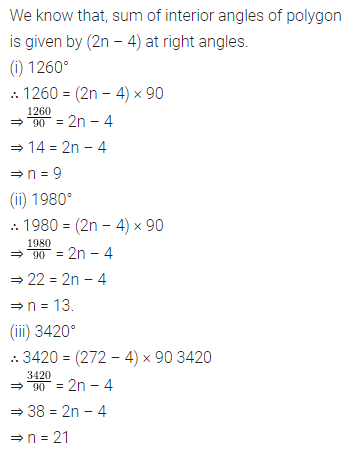Question 7.
If the angles of a pentagon are in the ratio 7 : 8 : 11 : 13 : 15, find the angles.
Solution:Question 8.
The angles of a pentagon are x°, (x – 10)°, (x + 20)°, (2x – 44)° and (2x – 70°) Calculate x.
Solution:Question 9.
The exterior angles of a pentagon are in ratio 1 : 2 : 3 : 4 : 5. Find all the interior angles of the pentagon.
Solution:Question 10.
In a quadrilateral ABCD, AB || DC. If ∠A : ∠D = 2:3 and ∠B : ∠C = ∠7 : 8, find the measure of each angle.
Solution:Question 11.
(i) x
(ii) ∠DAB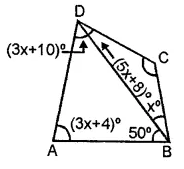Solution:Question 12.
Find the angle measure x in the following figures:Solution: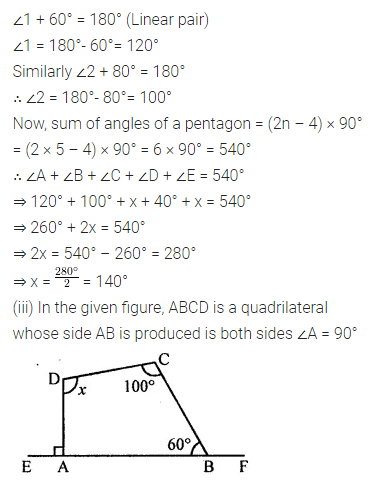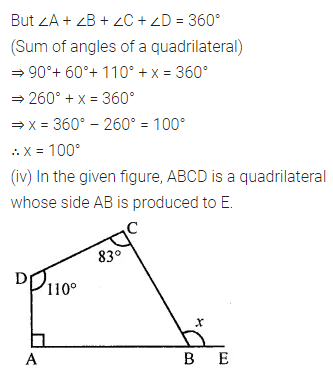Question 13.
(i) In the given figure, find x + y + z.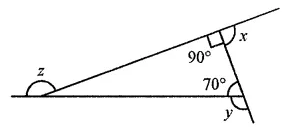(ii) In the given figure, find x + y + z + w.Solution: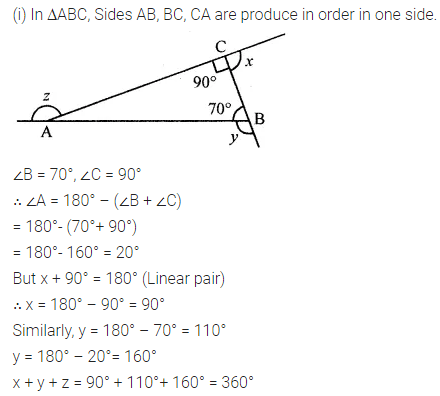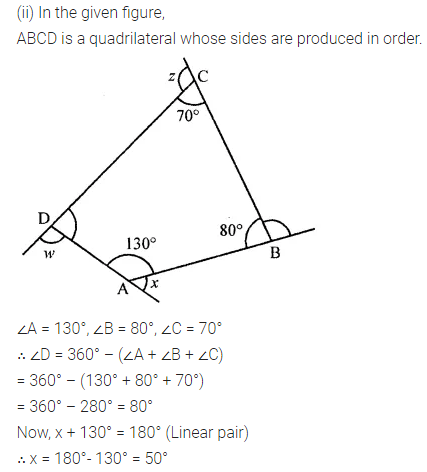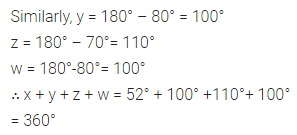Question 14.
A heptagon has three equal angles each of 120° and four equal angles. Find the size of equal angles.
Solution: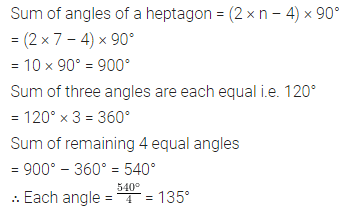Question 15.
The ratio between an exterior angle and the interior angle of a regular polygon is 1 : 5. Find
(i) the measure of each exterior angle
(ii) the measure of each interior angle
(iii) the number of sides in the polygon.
Solution: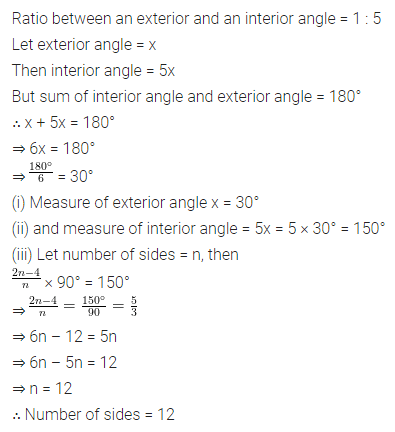Question 16.
Each interior angle of a regular polygon is double of its exterior angle. Find the number of sides in the polygon.
Solution: